# Forms Forms and Forms n What forms of

• Slides: 27Forms, Forms and Forms n What forms of quadratic equations have we learnt about so far? Standard Form y=ax 2+bx+c n Factored Form y=a(x-r)(x-s) nWhat useful info? n n n Remember, Standard form tells us some useful things: 1) Direction of opening 2) y intercept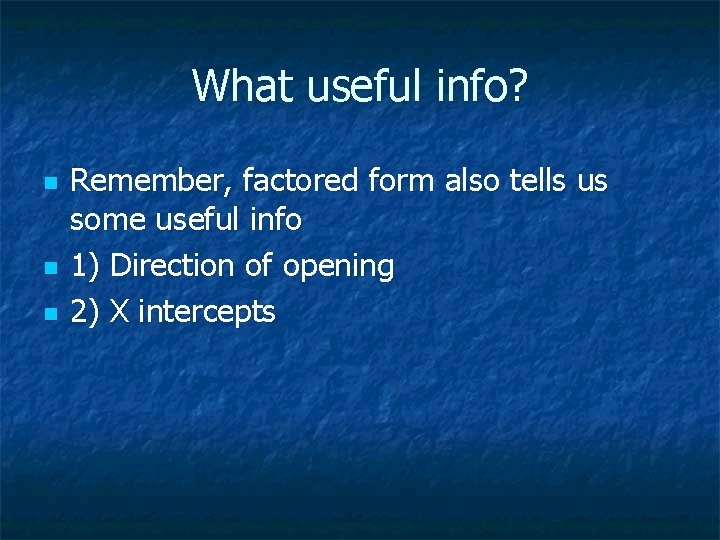What useful info? n n n Remember, factored form also tells us some useful info 1) Direction of opening 2) X interceptsNow there is another form to make your life more convenient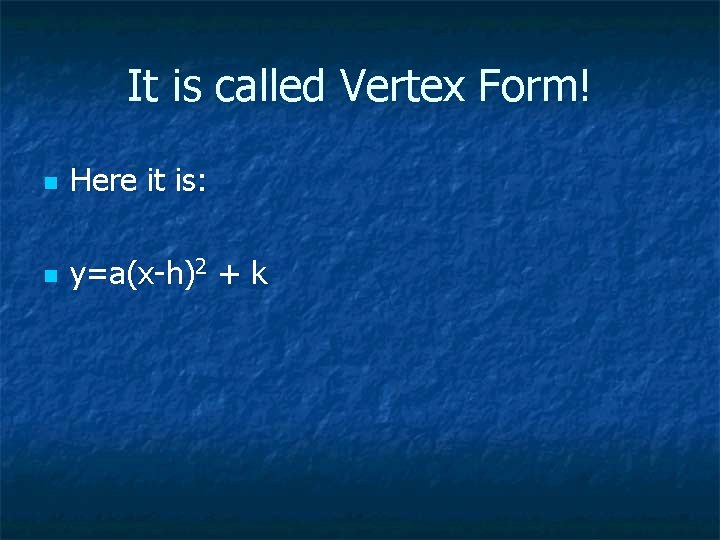It is called Vertex Form! n Here it is: n y=a(x-h)2 + kWhat does it all mean? n 2 y=a(x-h) Direction of opening +k X coordinate of vertex. Watch the sign Y coordinate of vertexCan you see why it might be useful? n n n Vertex form tells us 1) Direction of opening 2) Coordinates of the Vertex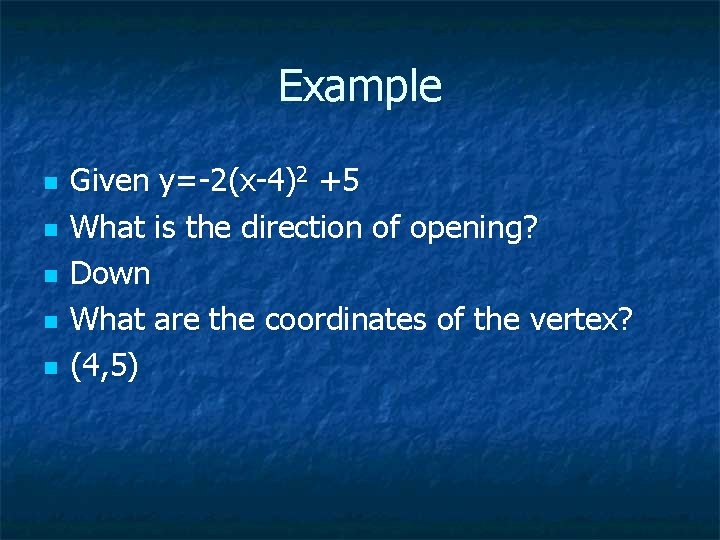Example n n n Given y=-2(x-4)2 +5 What is the direction of opening? Down What are the coordinates of the vertex? (4, 5)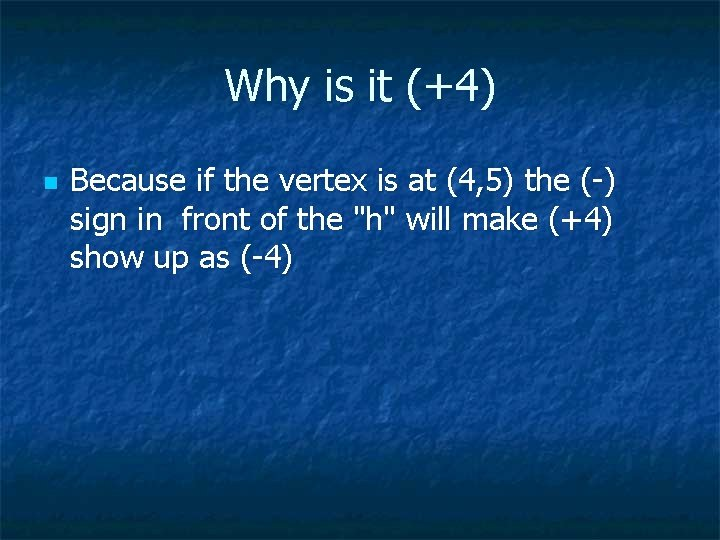Why is it (+4) n Because if the vertex is at (4, 5) the (-) sign in front of the "h" will make (+4) show up as (-4)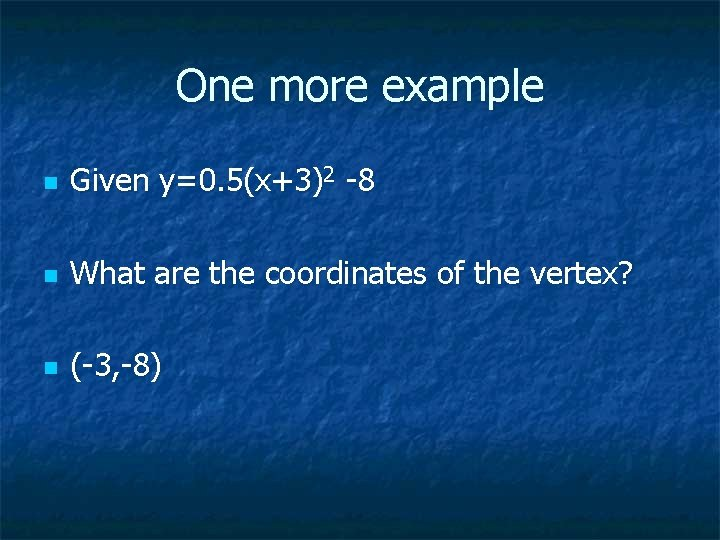One more example n Given y=0. 5(x+3)2 -8 n What are the coordinates of the vertex? n (-3, -8)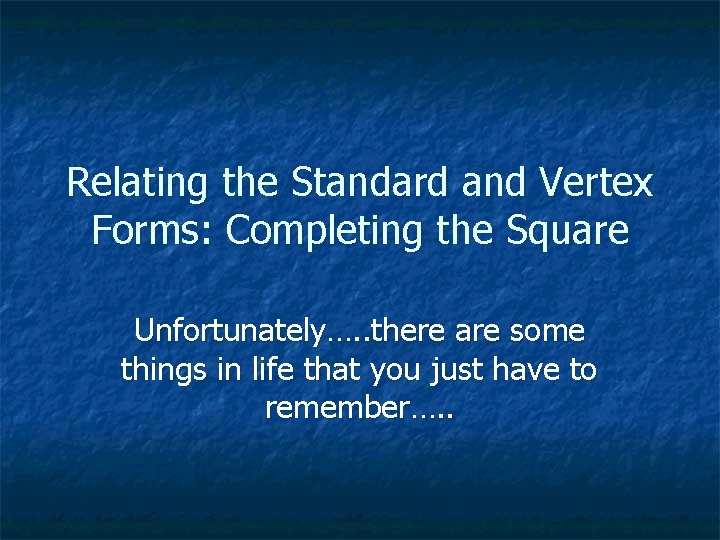Relating the Standard and Vertex Forms: Completing the Square Unfortunately…. . there are some things in life that you just have to remember…. .Step 1 n Insert brackets around the first two termsStep 2 n n Factor out any value in front of the x 2 In this case there is nothing to factor outStep 3 n Take Middle term divide by 2 & square it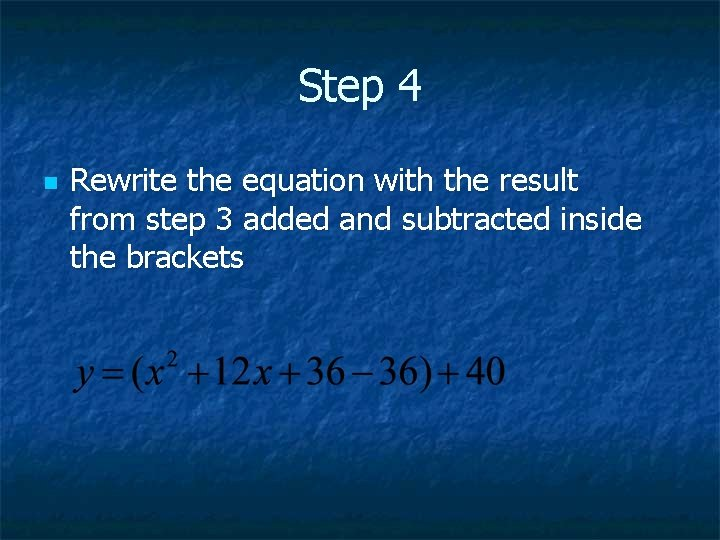Step 4 n Rewrite the equation with the result from step 3 added and subtracted inside the bracketsStep 5 n Bring the (-) term outside of the bracket remember to remultiply if necessary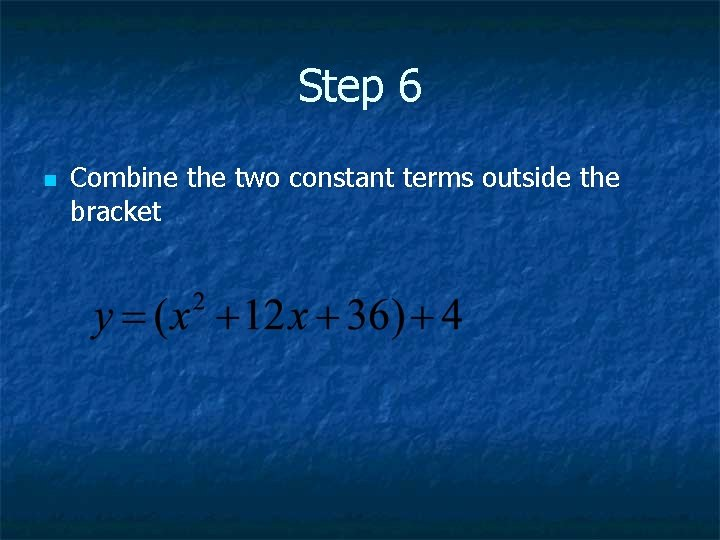Step 6 n Combine the two constant terms outside the bracketStep 7 n Factor trinomial inside the bracket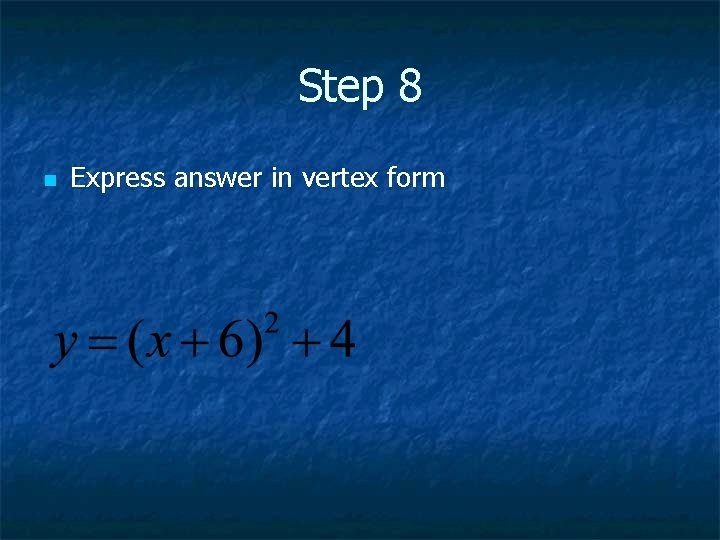Step 8 n Express answer in vertex form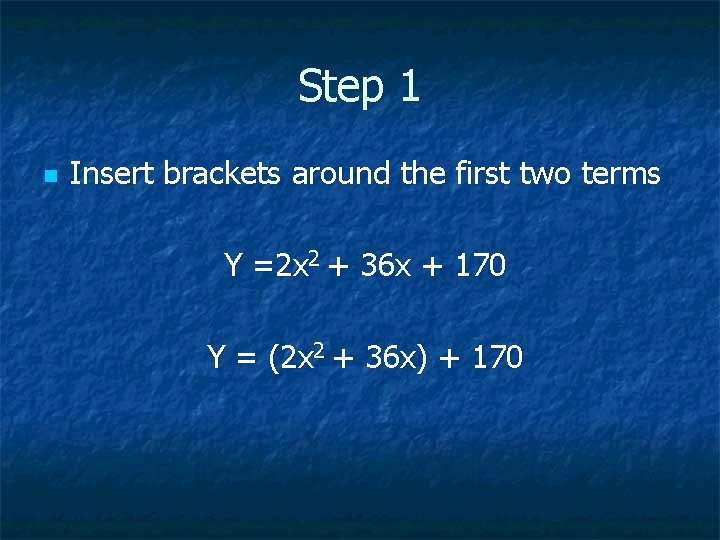Step 1 n Insert brackets around the first two terms Y =2 x 2 + 36 x + 170 Y = (2 x 2 + 36 x) + 170Step 2 n n Factor out any value in front of the x 2 In this case there is something to factor out Y = 2(x 2 + 18 x) + 170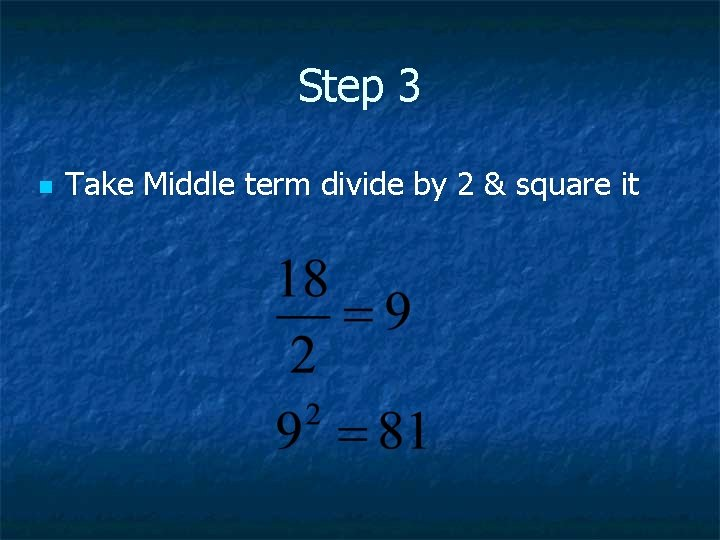Step 3 n Take Middle term divide by 2 & square it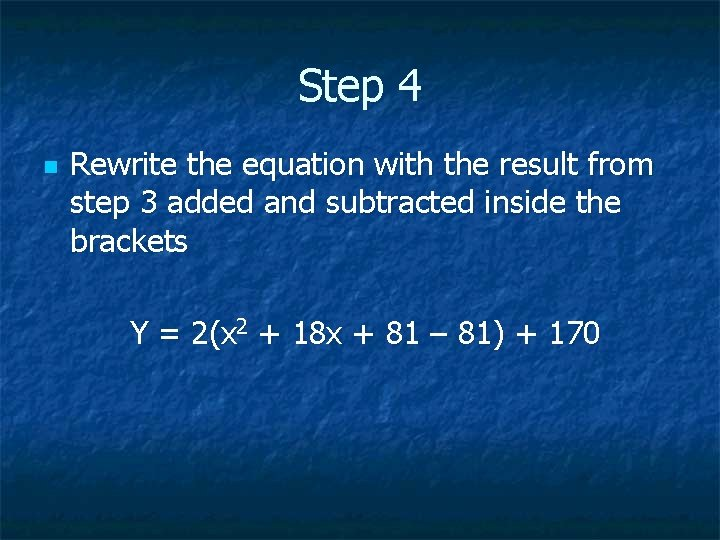Step 4 n Rewrite the equation with the result from step 3 added and subtracted inside the brackets Y = 2(x 2 + 18 x + 81 – 81) + 170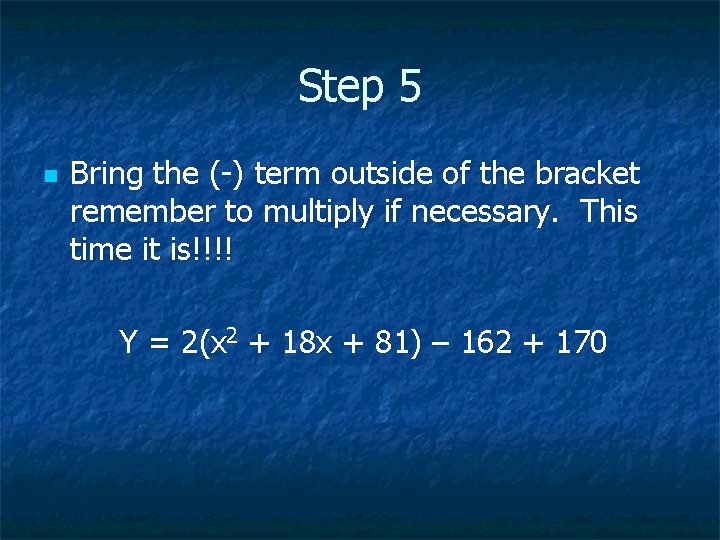Step 5 n Bring the (-) term outside of the bracket remember to multiply if necessary. This time it is!!!! Y = 2(x 2 + 18 x + 81) – 162 + 170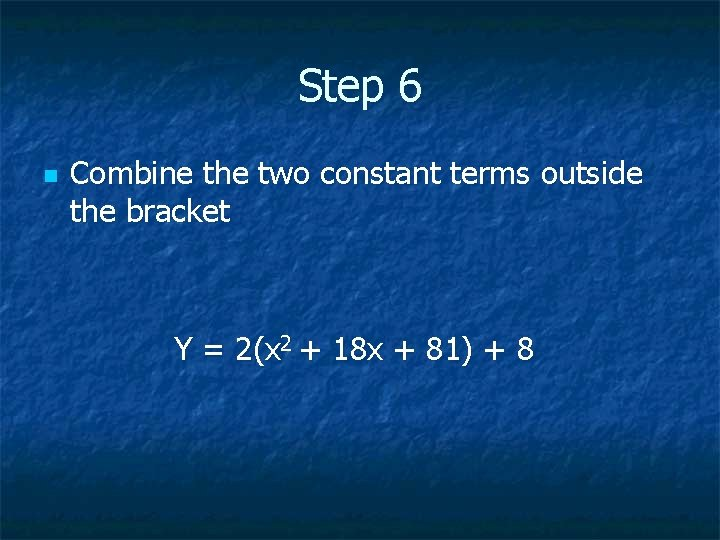Step 6 n Combine the two constant terms outside the bracket Y = 2(x 2 + 18 x + 81) + 8Step 7 n Factor trinomial inside the bracket Y = 2 (x + 9) ( x + 9) + 8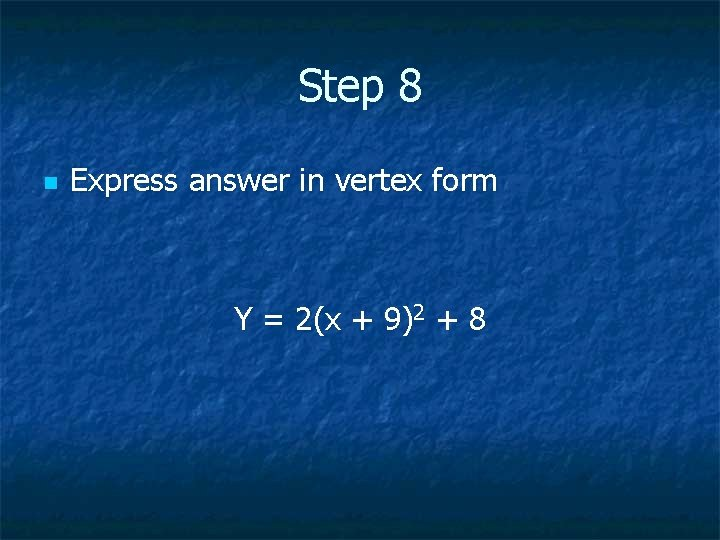Step 8 n Express answer in vertex form Y = 2(x + 9)2 + 8